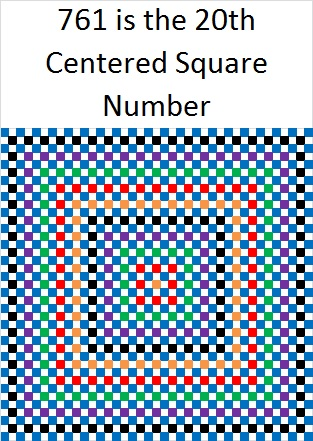# 761 and Level 1

• 761 is a prime number.
• Prime factorization: 761 is prime.
• The exponent of prime number 761 is 1. Adding 1 to that exponent we get (1 + 1) = 2. Therefore 761 has exactly 2 factors.
• Factors of 761: 1, 761
• Factor pairs: 761 = 1 x 761
• 761 has no square factors that allow its square root to be simplified. √761 ≈ 27.586228.How do we know that 761 is a prime number? If 761 were not a prime number, then it would be divisible by at least one prime number less than or equal to √761 ≈ 27.6. Since 761 cannot be divided evenly by 2, 3, 5, 7, 11, 13, 17, 19, or 23, we know that 761 is a prime number.

There will be a lot about squares in today’s post, and all of the clues in today’s puzzle are perfect squares, too:Print the puzzles or type the solution on this excel file: 10 Factors 2016-02-04

—————————————

Here’s more about the number 761:

Because 761 is a prime number, there is only one way to write it as the sum of consecutive numbers: 380 + 381 = 761.

However, because it is a centered square number, 761 can be written as the sum of consecutive square numbers: 20² + 19² = 761.And because it is the sum of two consecutive square numbers, it is also the hypotenuse of a primitive Pythagorean triple: 39-760-761 which was calculated using 20² – 19², 2(20)(19), 20² + 19².

Notice also that 20 + 19 = 39, ⌊39²/2⌋ = 760, and ⌈39²/2⌉ = 761. (Those peculiar parenthesis mean⌊round down to the closest whole number⌋ and ⌈round up to the closest whole number⌉.)

In the 39 x 39 graphic shown above there are 760 white squares and 761 squares with some color. Thus, looking at that graphic is one way to look at the primitive Pythagorean triple 39-760-761.

All of that gives us another way to know that 761 is a prime number: Since its last two digits divided by 4 leave a remainder of 1, and 20² + 19² = 761 with 20 and 19 having no common prime factors, 761 will be prime unless it is divisible by a prime number Pythagorean hypotenuse less than or equal to √761 ≈ 27.6. Since 761 is not divisible by 5, 13, or 17, we know that 761 is a prime number.

761 can also be expressed as the sum of three squares TEN ways:

• 27² + 4² + 4² = 761
• 26² + 9² + 2² = 761
• 26² + 7² + 6² = 761
• 25² + 10² + 6² = 761
• 24² + 13² + 4² = 761
• 24² + 11² + 8² = 761
• 23² + 14² + 6² = 761
• 22² + 14² + 9² = 761
• 21² + 16² + 8² = 761
• 19² + 16² + 12² = 761

761 is a palindrome in two different bases:

• 535 BASE 12; note that 5(144) + 3(12) + 5(1) = 761.
• 1I1 BASE 20 (I = 18 base 10); note that 1(400) + 18(20) + 1(1) = 761.

—————————————